Скачать презентацию Simulations and experimental study of DDT behind a

5eddb94bbc8fb8f1b2bcd36b090eff71.ppt

• Количество слайдов: 29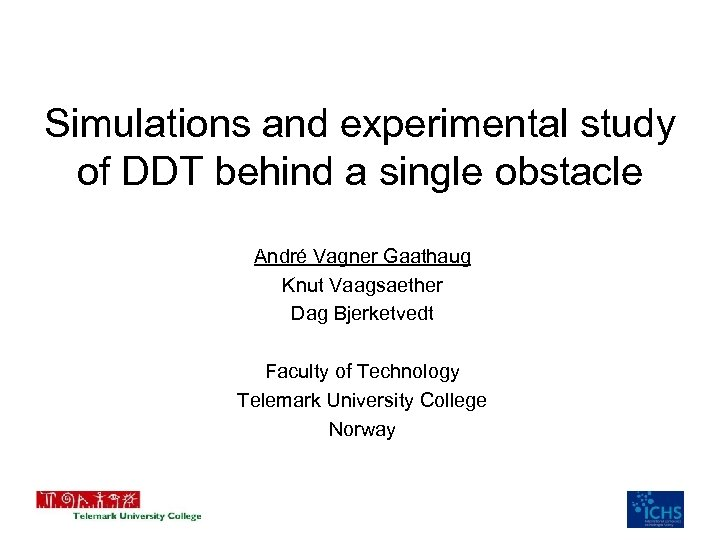Simulations and experimental study of DDT behind a single obstacle André Vagner Gaathaug Knut Vaagsaether Dag Bjerketvedt Faculty of Technology Telemark University College Norway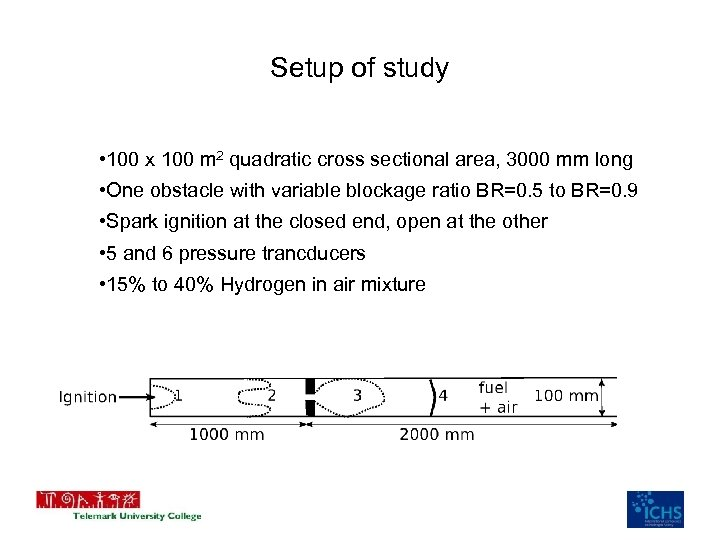Setup of study • 100 x 100 m 2 quadratic cross sectional area, 3000 mm long • One obstacle with variable blockage ratio BR=0. 5 to BR=0. 9 • Spark ignition at the closed end, open at the other • 5 and 6 pressure trancducers • 15% to 40% Hydrogen in air mixture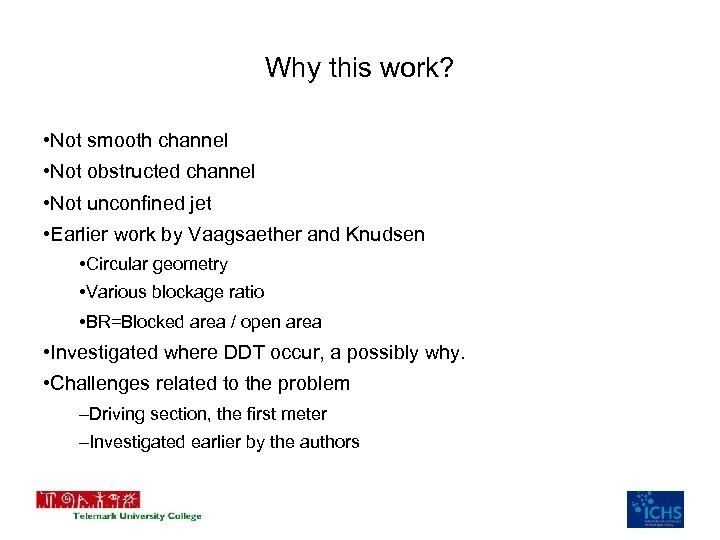Why this work? • Not smooth channel • Not obstructed channel • Not unconfined jet • Earlier work by Vaagsaether and Knudsen • Circular geometry • Various blockage ratio • BR=Blocked area / open area • Investigated where DDT occur, a possibly why. • Challenges related to the problem –Driving section, the first meter –Investigated earlier by the authors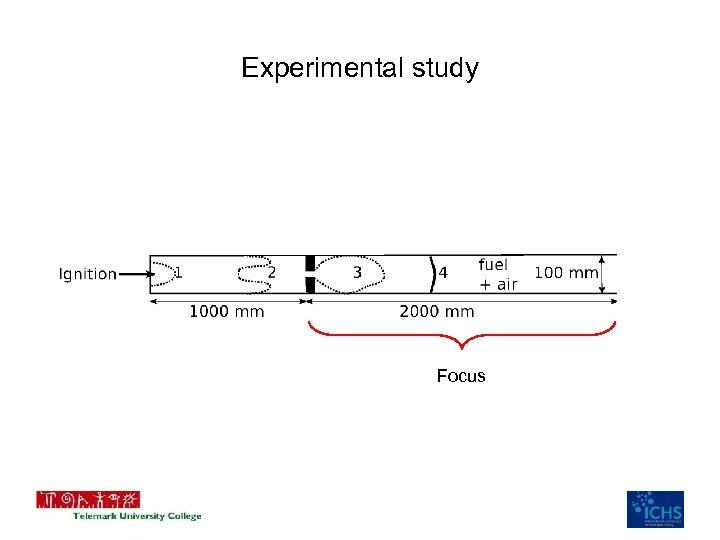Experimental study Focus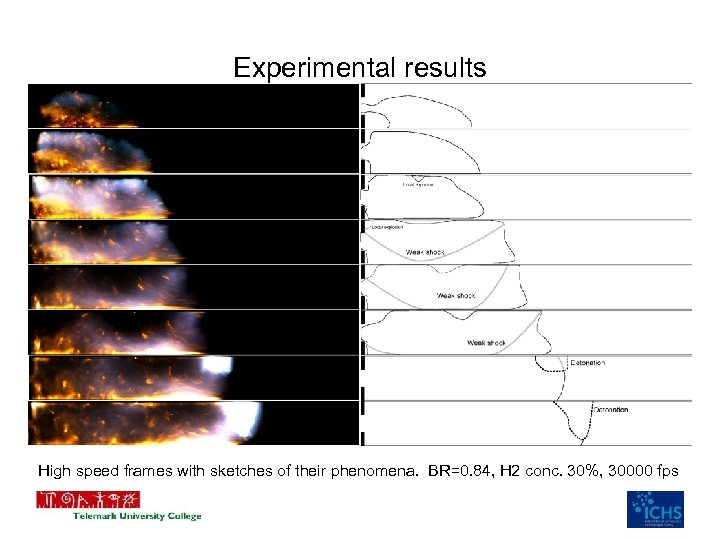Experimental results High speed frames with sketches of their phenomena. BR=0. 84, H 2 conc. 30%, 30000 fps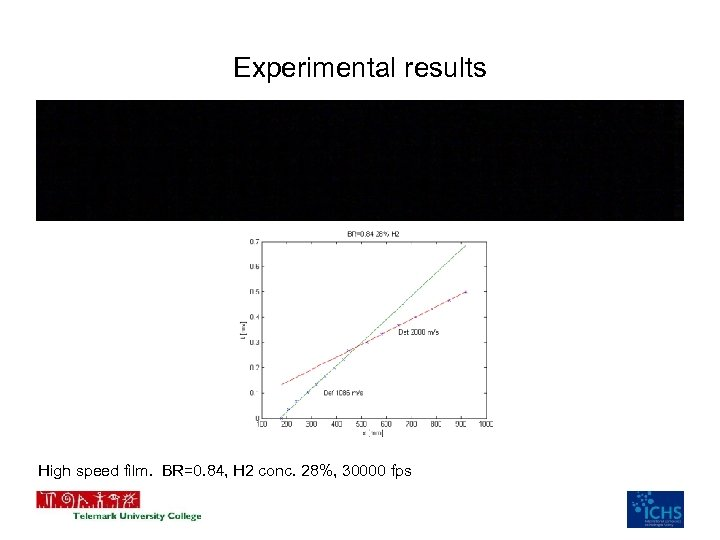Experimental results High speed film. BR=0. 84, H 2 conc. 28%, 30000 fps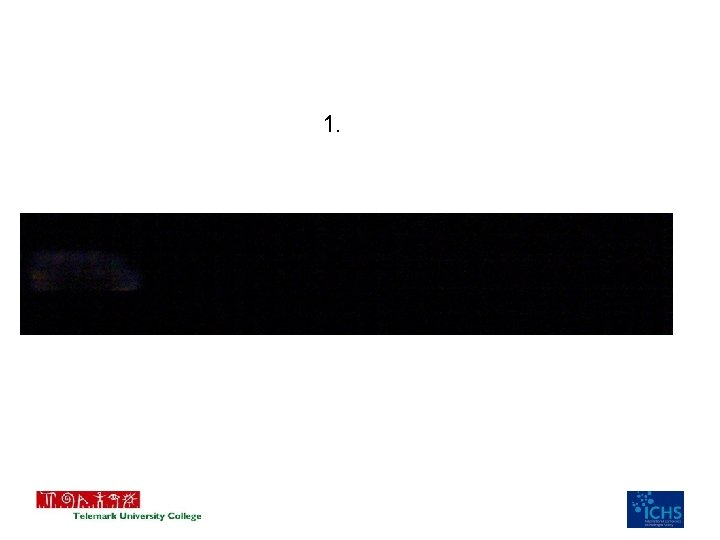1.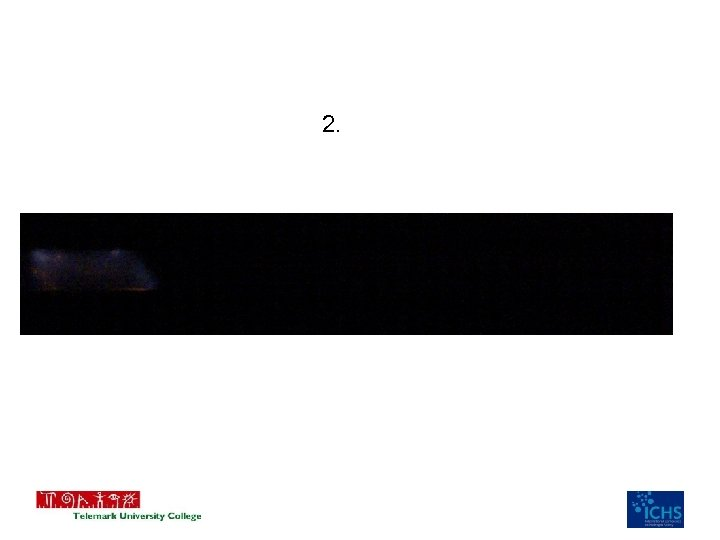2.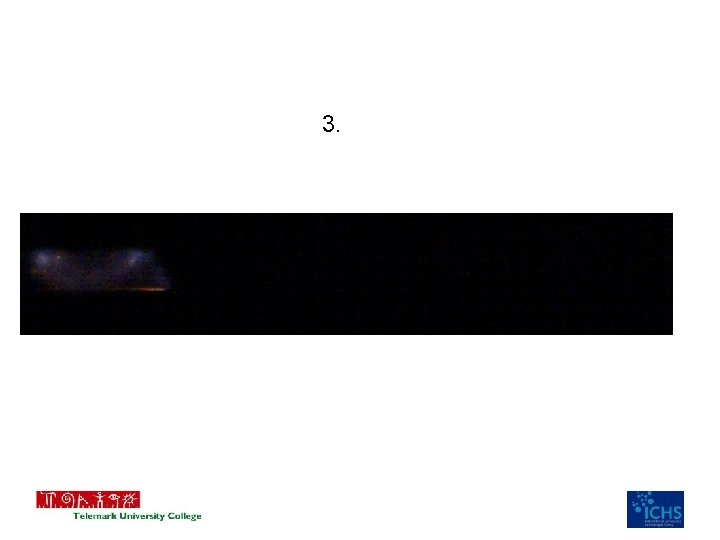3.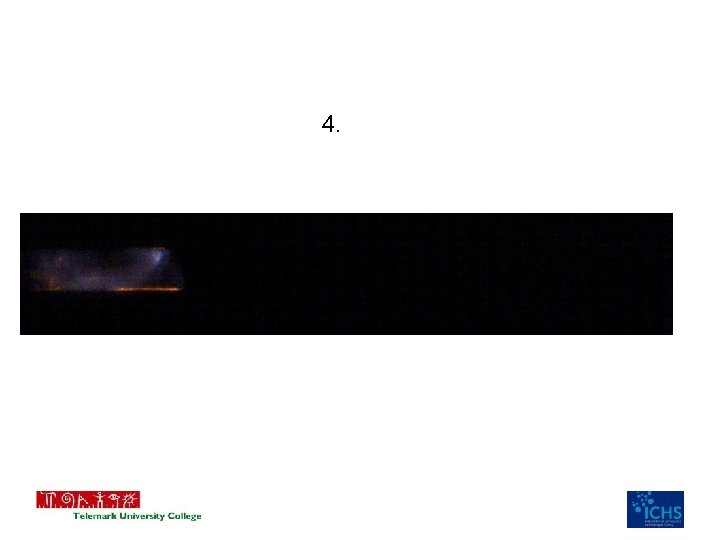4.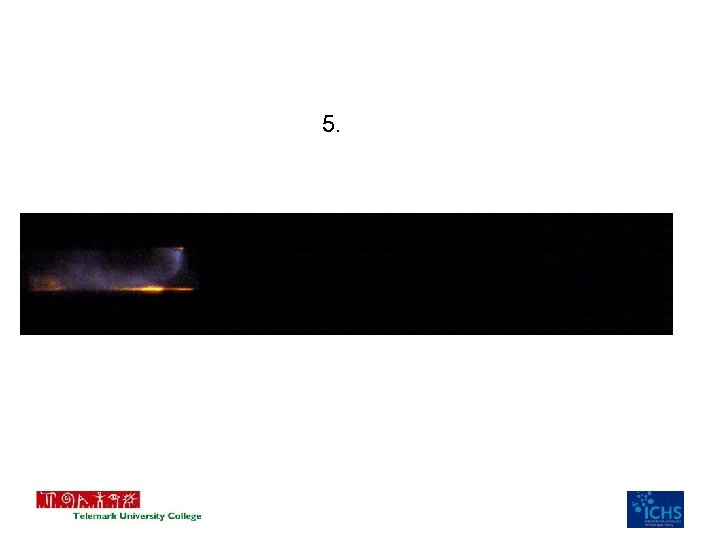5.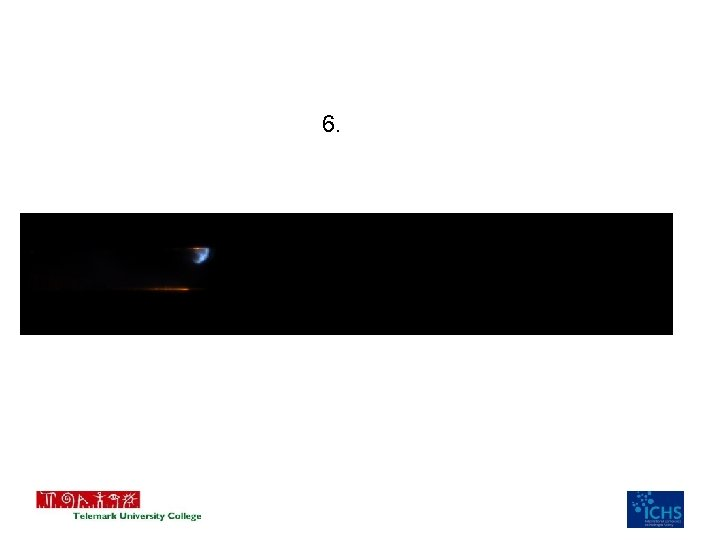6.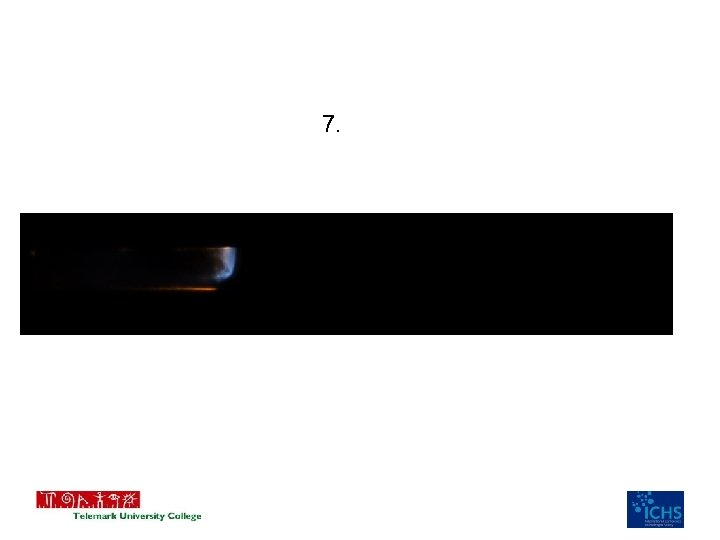7.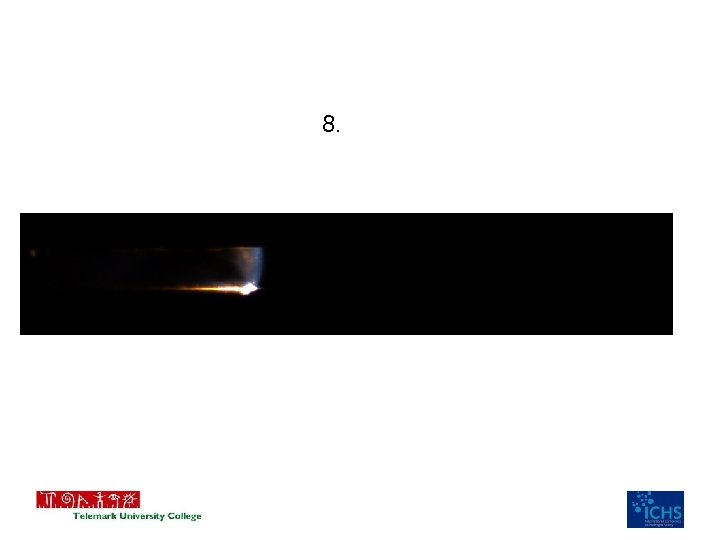8.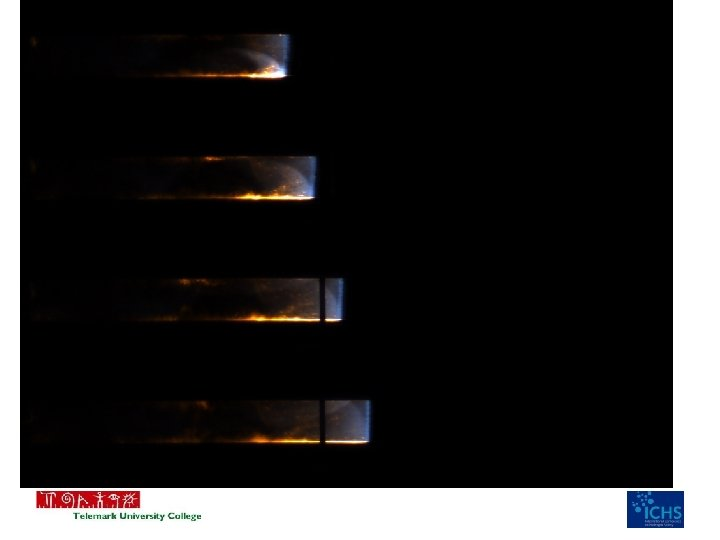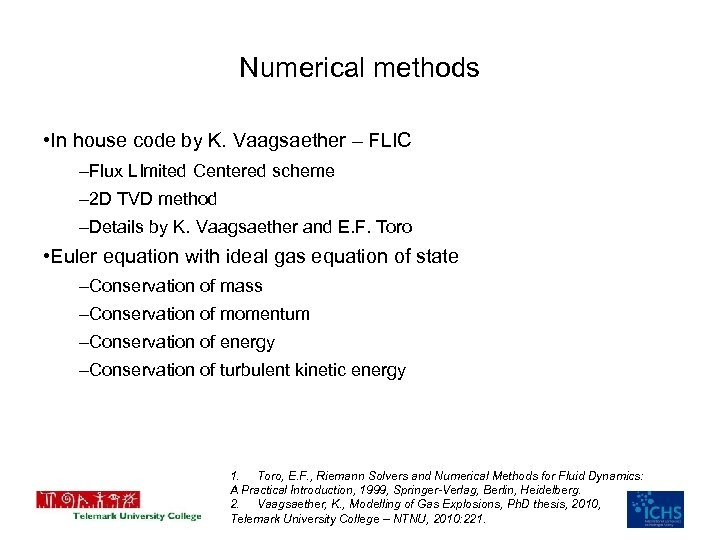Numerical methods • In house code by K. Vaagsaether – FLIC –Flux LImited Centered scheme – 2 D TVD method –Details by K. Vaagsaether and E. F. Toro • Euler equation with ideal gas equation of state –Conservation of mass –Conservation of momentum –Conservation of energy –Conservation of turbulent kinetic energy 1. Toro, E. F. , Riemann Solvers and Numerical Methods for Fluid Dynamics: A Practical Introduction, 1999, Springer-Verlag, Berlin, Heidelberg. 2. Vaagsaether, K. , Modelling of Gas Explosions, Ph. D thesis, 2010, Telemark University College – NTNU, 2010: 221.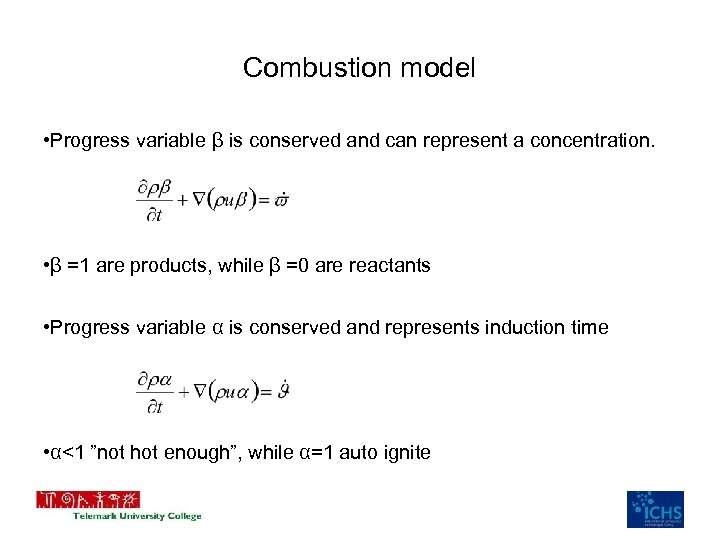Combustion model • Progress variable β is conserved and can represent a concentration. • β =1 are products, while β =0 are reactants • Progress variable α is conserved and represents induction time • α<1 ”not hot enough”, while α=1 auto ignite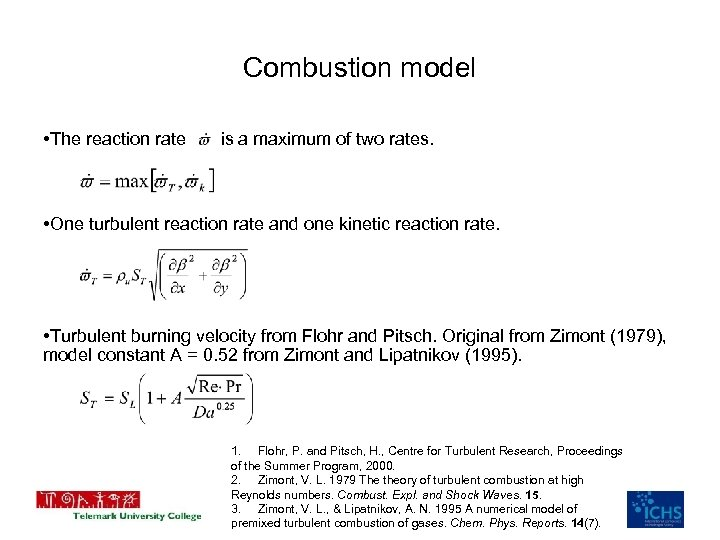Combustion model • The reaction rate is a maximum of two rates. • One turbulent reaction rate and one kinetic reaction rate. • Turbulent burning velocity from Flohr and Pitsch. Original from Zimont (1979), model constant A = 0. 52 from Zimont and Lipatnikov (1995). 1. Flohr, P. and Pitsch, H. , Centre for Turbulent Research, Proceedings of the Summer Program, 2000. 2. Zimont, V. L. 1979 The theory of turbulent combustion at high Reynolds numbers. Combust. Expl. and Shock Waves. 15. 3. Zimont, V. L. , & Lipatnikov, A. N. 1995 A numerical model of premixed turbulent combustion of gases. Chem. Phys. Reports. 14(7).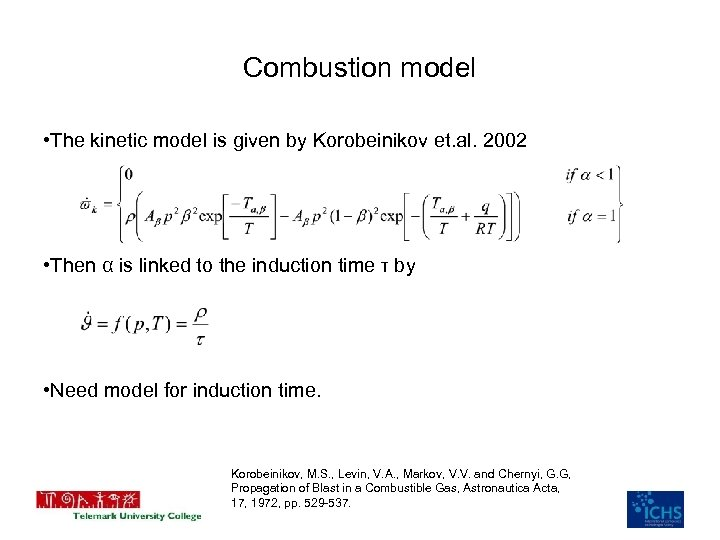Combustion model • The kinetic model is given by Korobeinikov et. al. 2002 • Then α is linked to the induction time τ by • Need model for induction time. Korobeinikov, M. S. , Levin, V. A. , Markov, V. V. and Chernyi, G. G, Propagation of Blast in a Combustible Gas, Astronautica Acta, 17, 1972, pp. 529 -537.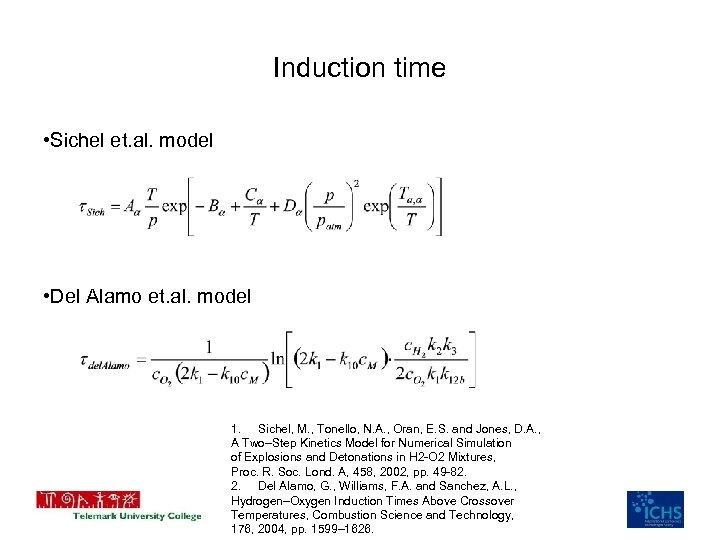Induction time • Sichel et. al. model • Del Alamo et. al. model 1. Sichel, M. , Tonello, N. A. , Oran, E. S. and Jones, D. A. , A Two–Step Kinetics Model for Numerical Simulation of Explosions and Detonations in H 2 -O 2 Mixtures, Proc. R. Soc. Lond. A, 458, 2002, pp. 49 -82. 2. Del Alamo, G. , Williams, F. A. and Sanchez, A. L. , Hydrogen–Oxygen Induction Times Above Crossover Temperatures, Combustion Science and Technology, 176, 2004, pp. 1599– 1626.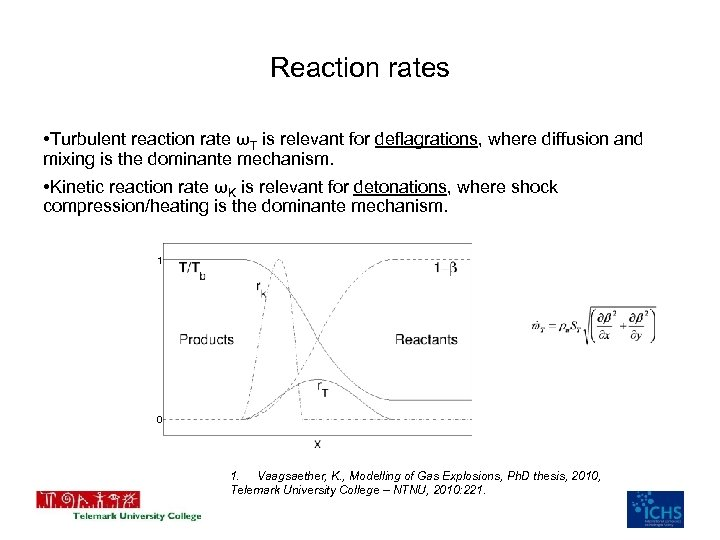Reaction rates • Turbulent reaction rate ωT is relevant for deflagrations, where diffusion and mixing is the dominante mechanism. • Kinetic reaction rate ωK is relevant for detonations, where shock compression/heating is the dominante mechanism. 1 0 1. Vaagsaether, K. , Modelling of Gas Explosions, Ph. D thesis, 2010, Telemark University College – NTNU, 2010: 221.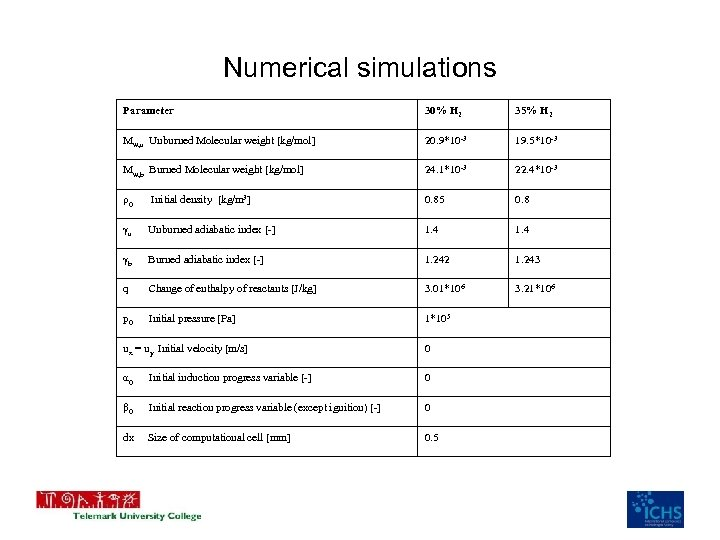Numerical simulations Parameter 30% H 2 35% H 2 Mw, u Unburned Molecular weight [kg/mol] 20. 9*10 -3 19. 5*10 -3 Mw, b Burned Molecular weight [kg/mol] 24. 1*10 -3 22. 4*10 -3 ρ0 Initial density [kg/m 3] 0. 85 0. 8 γu Unburned adiabatic index [-] 1. 4 γb Burned adiabatic index [-] 1. 242 1. 243 q Change of enthalpy of reactants [J/kg] 3. 01*106 3. 21*106 p 0 Initial pressure [Pa] 1*105 ux = uy Initial velocity [m/s] 0 α 0 Initial induction progress variable [-] 0 β 0 Initial reaction progress variable (except ignition) [-] 0 dx Size of computational cell [mm] 0. 5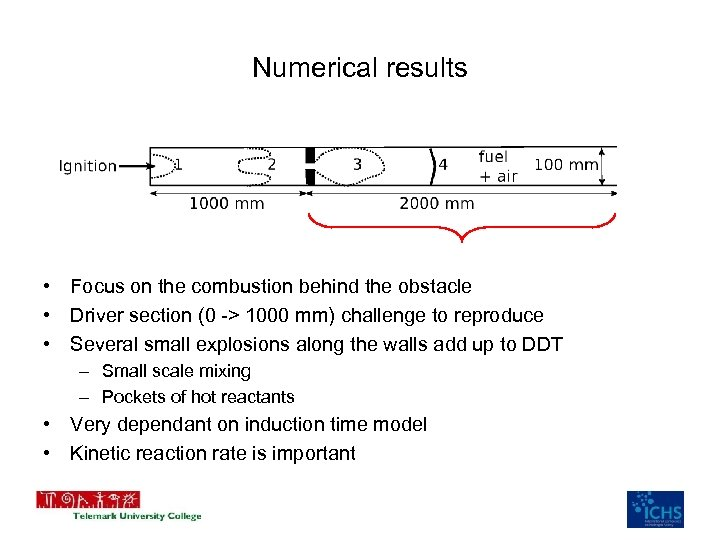Numerical results • Focus on the combustion behind the obstacle • Driver section (0 -> 1000 mm) challenge to reproduce • Several small explosions along the walls add up to DDT – Small scale mixing – Pockets of hot reactants • Very dependant on induction time model • Kinetic reaction rate is important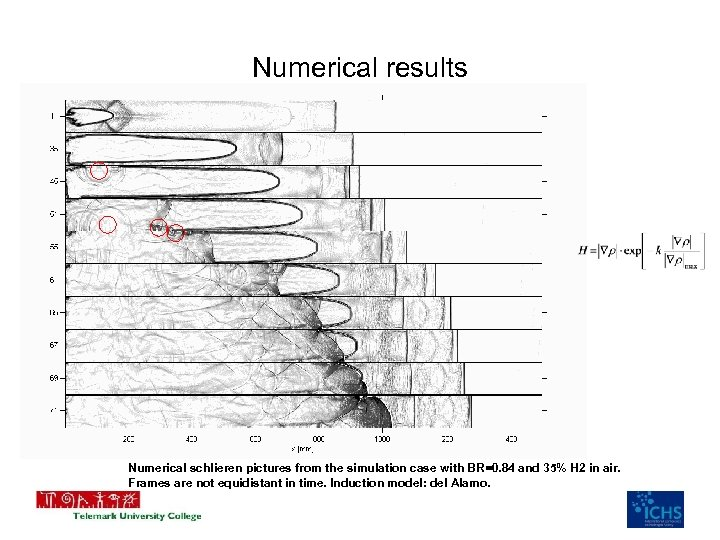Numerical results Numerical schlieren pictures from the simulation case with BR=0. 84 and 35% H 2 in air. Frames are not equidistant in time. Induction model: del Alamo.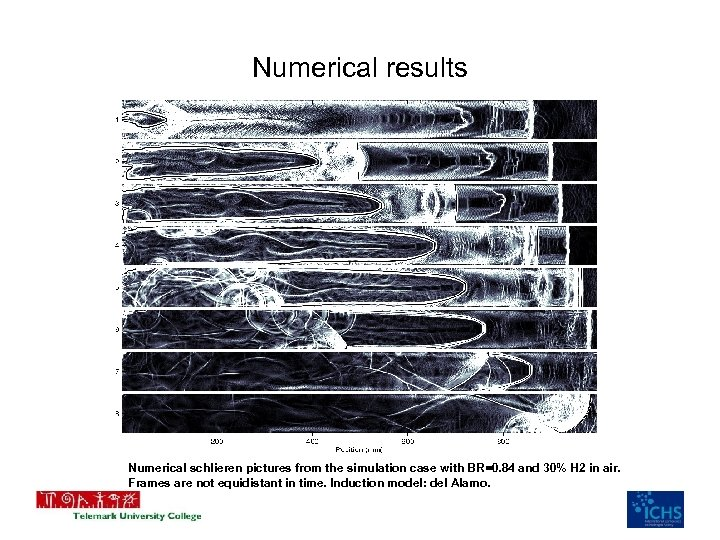Numerical results Numerical schlieren pictures from the simulation case with BR=0. 84 and 30% H 2 in air. Frames are not equidistant in time. Induction model: del Alamo.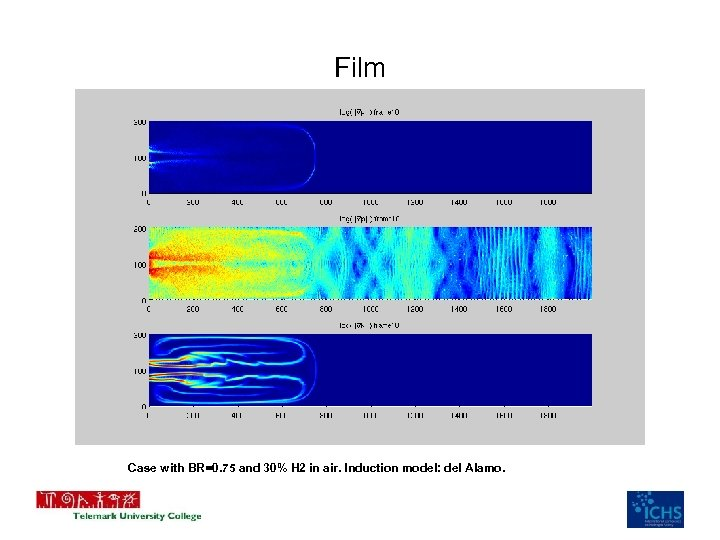Film Case with BR=0. 75 and 30% H 2 in air. Induction model: del Alamo.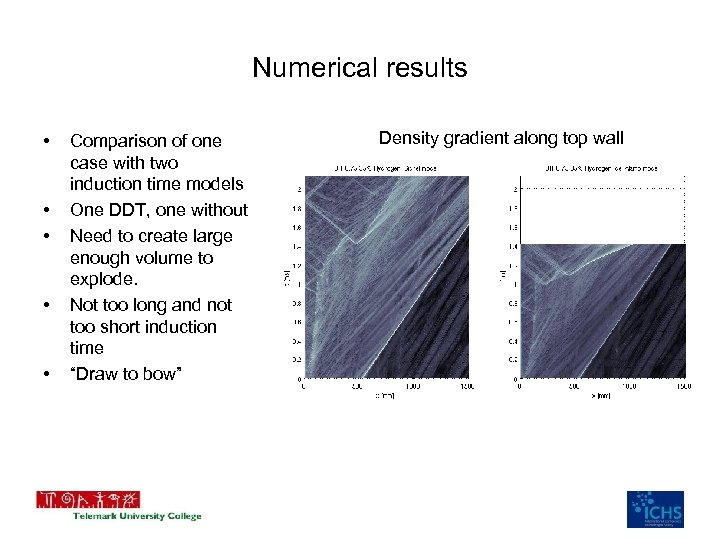Numerical results • • • Comparison of one case with two induction time models One DDT, one without Need to create large enough volume to explode. Not too long and not too short induction time “Draw to bow” Density gradient along top wall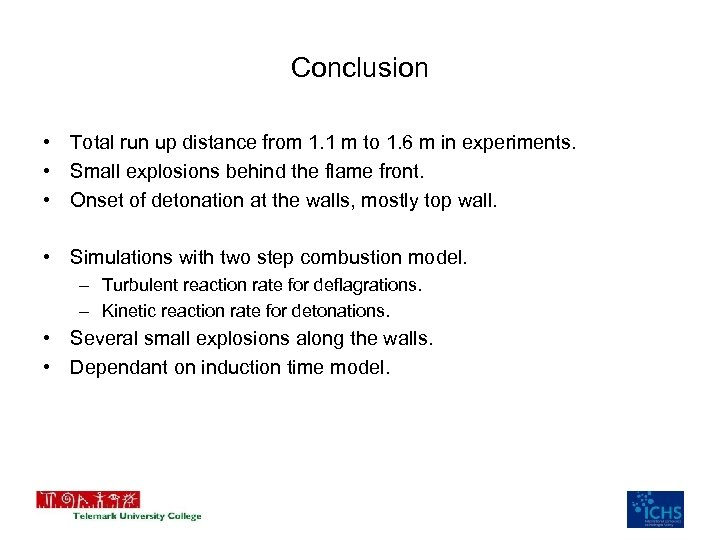Conclusion • Total run up distance from 1. 1 m to 1. 6 m in experiments. • Small explosions behind the flame front. • Onset of detonation at the walls, mostly top wall. • Simulations with two step combustion model. – Turbulent reaction rate for deflagrations. – Kinetic reaction rate for detonations. • Several small explosions along the walls. • Dependant on induction time model.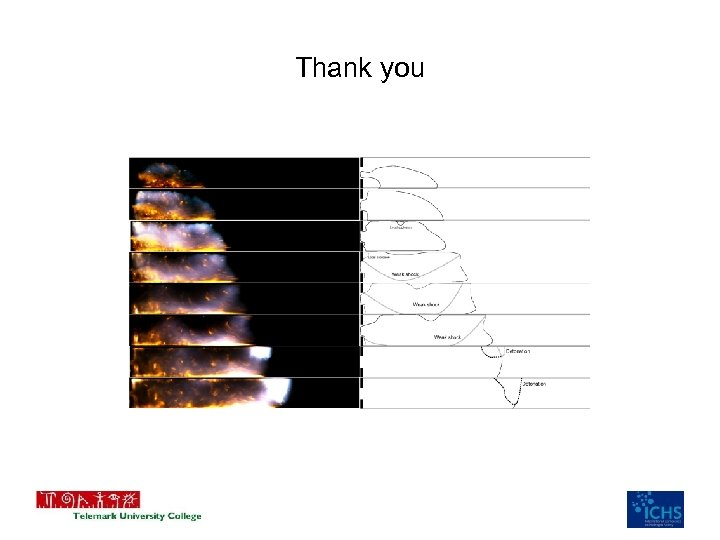Thank you